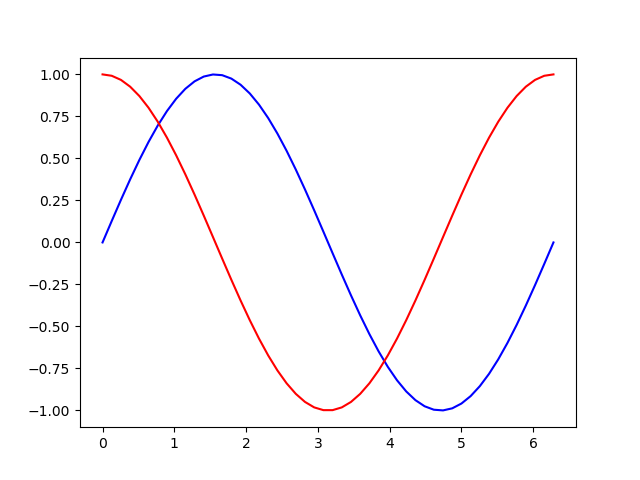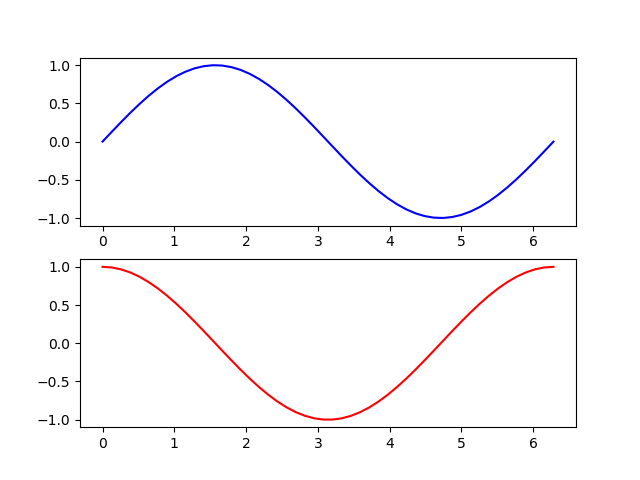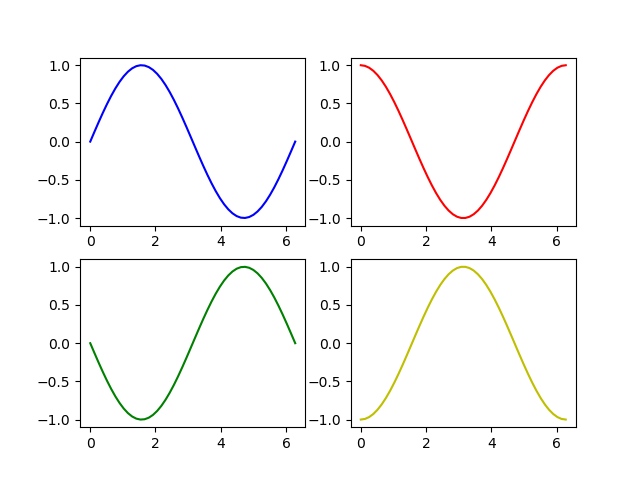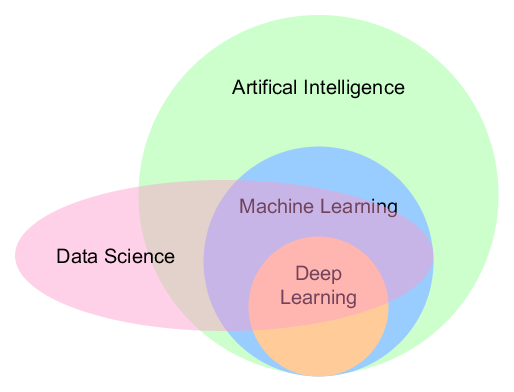## Distributing your own package on PyPi – Part 2

In Distributing your own package on PyPi I wrote about my first package on PyPI. Here are some refinements aka lessons learned:

## Project Description on PyPI

I wondered why the project description on PyPi was empty. Solution: You need a long_description. If You already have a README.md, you can read it into a string and use this as the description.

But you have to add long_description_content_type=’text/markdown’ as well.

```from setuptools import setup

from os import path
this_directory = path.abspath(path.dirname(__file__))
with open(path.join(this_directory, 'README.md'), encoding='utf-8') as f:

setup(
version='0.6',
author='Joern Boegeholz',
author_email='boegeholz.joern@gmail.com',
description='Django-style URL handling for Flask',
long_description=long_description,
long_description_content_type='text/markdown',
)```

## Dependencies of your Package

If your package relies on the usage of other python packages you should add them to your setup.py as well via install_requires.

```setup(
version='0.6',
author='Joern Boegeholz',
author_email='boegeholz.joern@gmail.com',
description='Django-style url handling for Flask',
)```

## Introduction to matplotlib – Part 2

When you finished reading part 1 of the introduction you might have wondered how to draw more than one line or curve into on plot. I will show you now.

To make it a bit more interesting we generate two functions: sine and cosine. We generate our x-values with numpy’s linspace function

```import numpy as np
import matplotlib.pyplot as plt

x = np.linspace(0, 2*np.pi)

sin = np.sin(x)
cos = np.cos(x)

plt.plot(x, sin, color='b')
plt.plot(x, cos, color='r')
plt.show()```

You can plot two or more curves by repeatedly calling the plot method.That’s fine as long as the individual plots share the same axis-description and values.

## Subplots

```fig = plt.figure()
p1 = fig.add_subplot(2, 1, 1)
p2 = fig.add_subplot(2, 1, 2)
p1.plot(x, sin, c='b')
p2.plot(x, cos, c='r'```

The add_subplot method allows us to put many plots into one “parent” plot aka figure. The arguments are (number_of_rows, number_of_columns, place in the matrix) So in this example we have 2 rows in 1 column, sine is in first, cosine in second position:when you have a 2 by 2 matrix it is counted from columns to row

```fig = plt.figure()
p1.plot(x, sin, c='b')
p2.plot(x, cos, c='r')
p3.plot(x, -sin, c='g')
p4.plot(x, -cos, c='y')```The code is available as a Jupyter Notebook on my github

## Python datetime and format

One of the things I always forget is date and time in Python.
So message to myself:

the strftime method is used for formatting (string_format_time)

```import datetime
start_date = datetime.datetime.now()
DATE_FORMAT = '%d/%m/%Y %H:%M'
print(start_date.strftime(DATE_FORMAT))```

Cheatsheet

## Python3: ChainMap

Since Python 3.3 You can chain dictionaries which contain the same key in a prioritized order:

```from collections import ChainMap

prio_1 = {"param_1": "foo"}
prio_2 = {"param_1": "foobar", "param_2": "bar"}

combined = ChainMap(prio_1, prio_2)

print(combined["param_1"]) # outputs 'foo'
print(combined["param_2"]) # outputs 'bar'```

## Celebration Grid

Everyone is talking about error culture and that companies should embrace it. Jurgen Appelo sheds some light on this topic by bisecting the term “error” and introducing a tool to celebrate the right kind of errors.Continue reading “Celebration Grid”

## SQL – NULL-safe equals operator

<=> is the NULL-safe equals operator

`SELECT * FROM reports WHERE NOT driver <=> user_id`

## 15 Steps for successful Bootstrapping – Part 2

this is the second part to Bootstrapping – Part 1

## Time to Cash – When do I get my money?

More important than “time to market” (when can I offer my product on the market) is “time to cash”: when do I get the money for my service?
For a successful bootstrapping, it makes no sense to sell and sell and to only bill once a year. Continue reading “15 Steps for successful Bootstrapping – Part 2”

## Test coverage

I wanted to analyze my python package flaskurls for test coverage. this is how you can do it:

```pipenv install pytest-cov
```----------- coverage: platform win32, python 3.6.5-final-0 -----------
Name                              Stmts   Miss  Cover
-----------------------------------------------------
flask_url_mapping\__init__.py         1      0   100%
-----------------------------------------------------
TOTAL                                74      0   100%

========================== 10 passed in 0.60 seconds ==========================
```

## 10 things I didn’t know about Data Science a year ago

In my article My personal road map for learning data science in 2018 I wrote about how I try to tackle the data science knowledge sphere. Due to the fact that 2018 is slowly coming to an end I think it is time for a little wrap up.

What are the things I learned about Data Science in 2018? Here we go:

## 1. The difference between Data Science, Machine Learning, Deep Learning and AIContinue reading “10 things I didn’t know about Data Science a year ago”